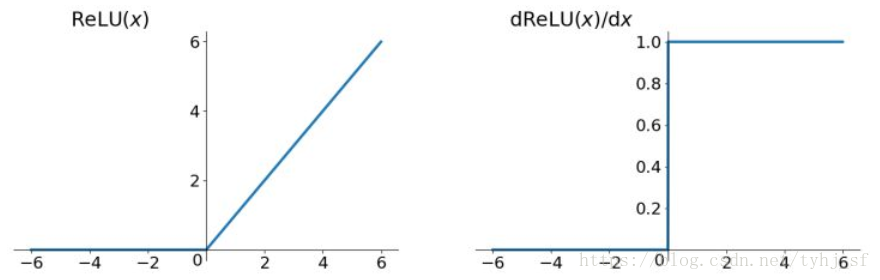# 1：什么是激活函数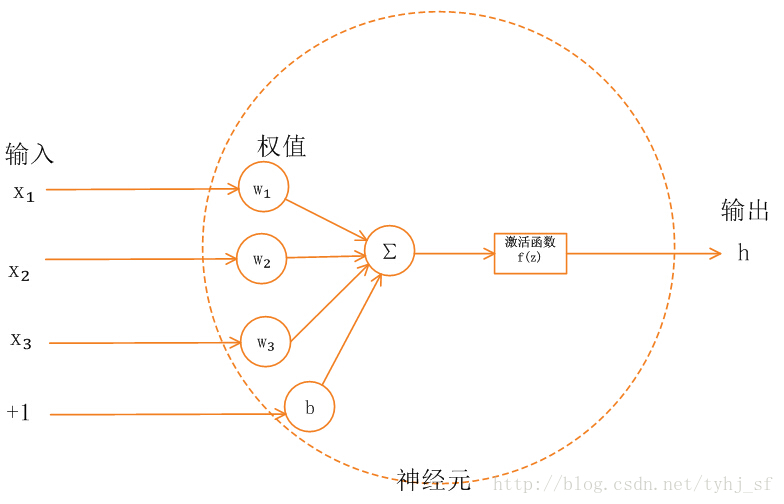# 2：为什么要激活函数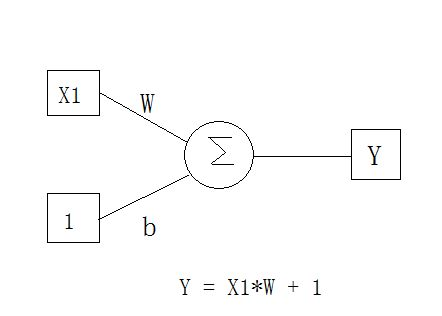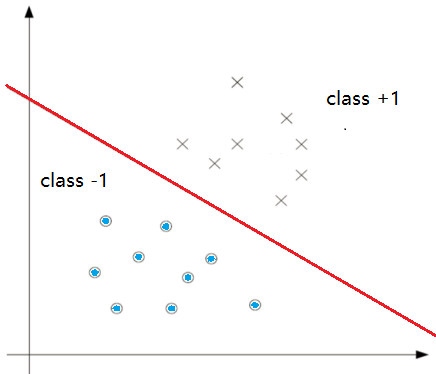# 3：哪些激活函数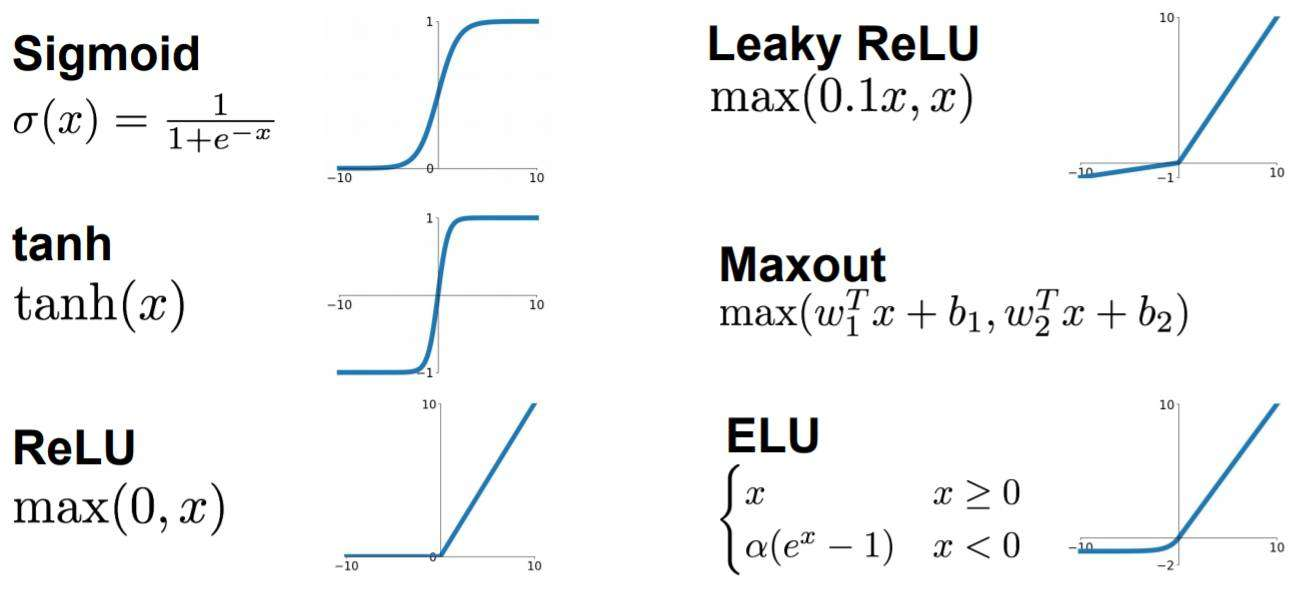• Sigmoid函数

Sigmoid函数只会输出0到1之间的正数，当输入无穷小时，输出为0，输入无穷大时，输出为1。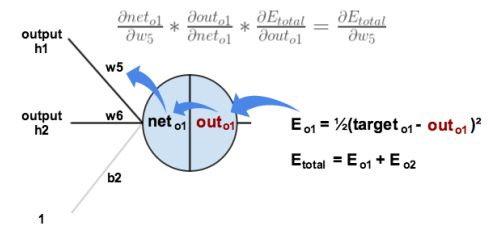$out_{o1}=Sigmoid(net_{o1})$

$$\frac{\partial E_{o1}}{\part w_5} = \frac{\part E_{o1}}{\part out_{o1}}* \frac{\part out_{o1}}{\part net_{o1}}* \frac{\part net_{o1}}{\part w_5}$$

$$\frac{\part g(z)}{\part z} = g(z)(1-g(z))$$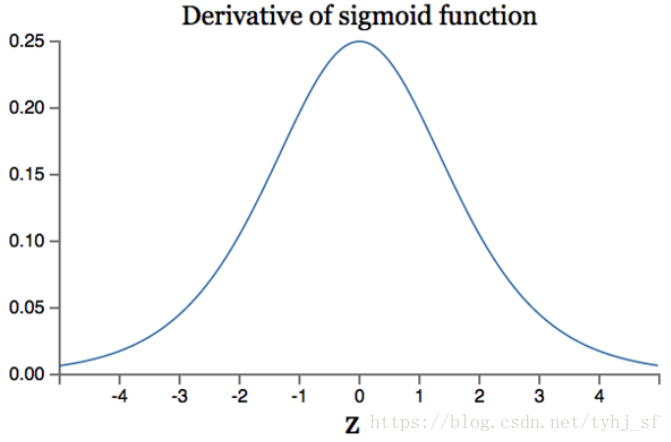• tanh函数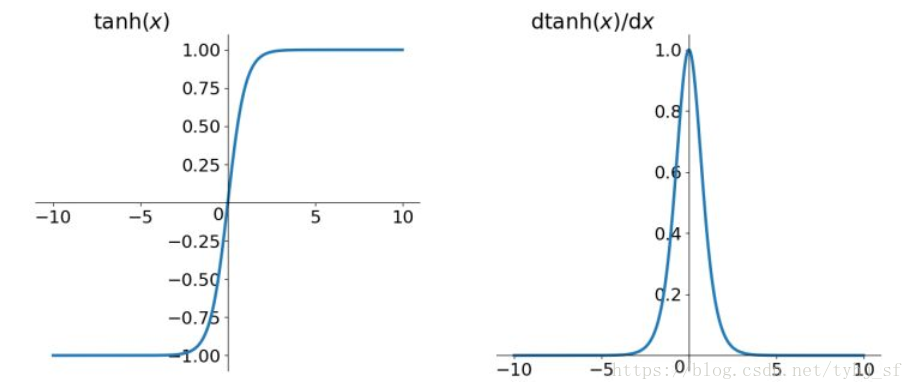• Relu函数